Publicité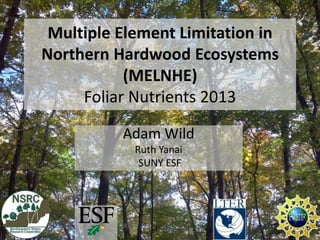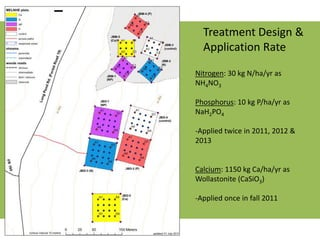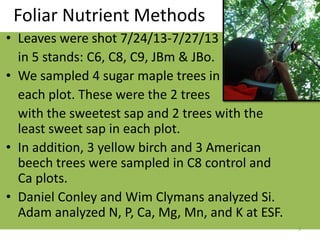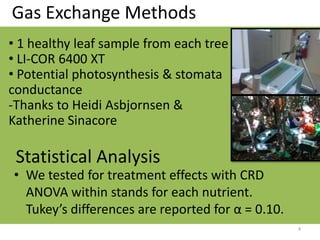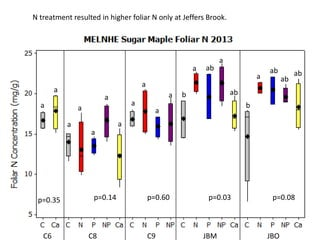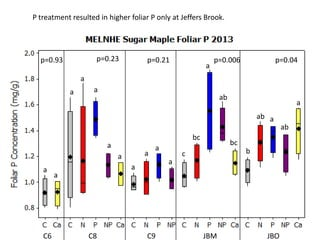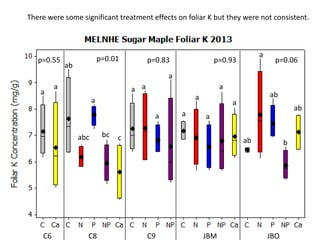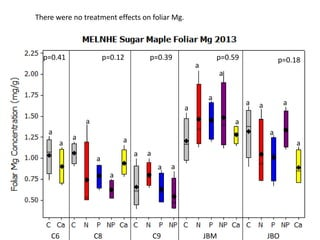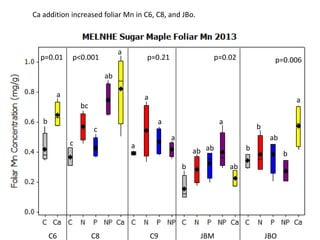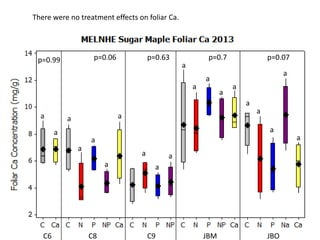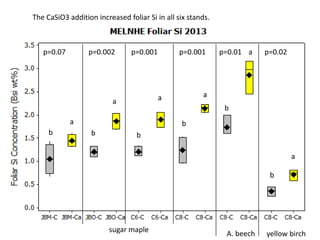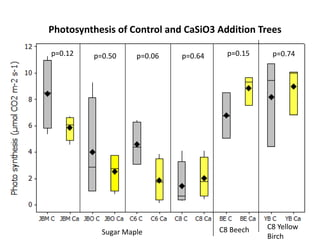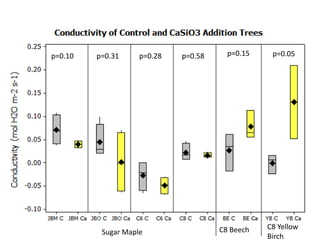1 sur 13
Publicité

### HBCOS-Wild-042214

1. Multiple Element Limitation in Northern Hardwood Ecosystems (MELNHE) Foliar Nutrients 2013 Adam Wild Ruth Yanai SUNY ESF
2. Nitrogen: 30 kg N/ha/yr as NH4NO3 Phosphorus: 10 kg P/ha/yr as NaH2PO4 -Applied twice in 2011, 2012 & 2013 Calcium: 1150 kg Ca/ha/yr as Wollastonite (CaSiO3) -Applied once in fall 2011 Treatment Design & Application Rate
3. Foliar Nutrient Methods • Leaves were shot 7/24/13-7/27/13 in 5 stands: C6, C8, C9, JBm & JBo. • We sampled 4 sugar maple trees in each plot. These were the 2 trees with the sweetest sap and 2 trees with the least sweet sap in each plot. • In addition, 3 yellow birch and 3 American beech trees were sampled in C8 control and Ca plots. • Daniel Conley and Wim Clymans analyzed Si. Adam analyzed N, P, Ca, Mg, Mn, and K at ESF. 3
4. Gas Exchange Methods 4 • We tested for treatment effects with CRD ANOVA within stands for each nutrient. Tukey’s differences are reported for α = 0.10. Statistical Analysis • 1 healthy leaf sample from each tree • LI-COR 6400 XT • Potential photosynthesis & stomata conductance -Thanks to Heidi Asbjornsen & Katherine Sinacore
5. C6 C8 C9 JBM JBO p=0.08p=0.03p=0.60p=0.14p=0.35 b a ab ab ab a b ab ab a a a a a a a a a a a a N treatment resulted in higher foliar N only at Jeffers Brook.
6. C6 C8 C9 JBM JBO p=0.04p=0.006p=0.21p=0.23p=0.93 b ab ab a a a ab c bc bc a a a a a a a a a a a P treatment resulted in higher foliar P only at Jeffers Brook.
7. C6 C8 C9 JBM JBO p=0.06p=0.93p=0.83p=0.01p=0.55 ab a ab ab b a a a a a a a a a a ab cabc bc a a There were some significant treatment effects on foliar K but they were not consistent.
8. C6 C8 C9 JBM JBO p=0.18p=0.59p=0.39p=0.12p=0.41 a a a a a a a a a a a a a a a a a a a a a There were no treatment effects on foliar Mg.
9. C6 C8 C9 JBM JBO p=0.006p=0.02p=0.21p<0.001p=0.01 a ab b b b a b ab ab ab a a a a a ab bc c c a b Ca addition increased foliar Mn in C6, C8, and JBo.
10. C6 C8 C9 JBM JBO p=0.07 a a a a a p=0.7p=0.63p=0.06p=0.99 a a a a a a a a a a a a aa a There were no treatment effects on foliar Ca.
11. sugar maple A. beech yellow birch p=0.001 p=0.001 p=0.01 p=0.02p=0.07 p=0.002 b a b a b a b a b a b a The CaSiO3 addition increased foliar Si in all six stands.
12. p=0.15 p=0.74p=0.64 C8 Beech C8 Yellow Birch Sugar Maple p=0.06p=0.50p=0.12 Photosynthesis of Control and CaSiO3 Addition Trees
13. p=0.15 p=0.05p=0.28 C8 Beech C8 Yellow Birch Sugar Maple p=0.58p=0.10 p=0.31

### Notes de l'éditeur

1. We have stands at 3 sites, all in the WM of NH. At JB and HB, we have 2 stands, one mid-aged and one old.The treatment plots include Control, N, P, N+P, and sometimes, where we had room, a Ca treatment plot.Most of the plots are 50x50 m, except in some of the young stands, 30x30.
Publicité